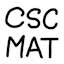#My university courses

I sometimes get asked about what courses I took at postsecondary school. So I’m publishing this list, hoping that it can start conversations on topics I’m familiar with.

I did a major in computer science and a minor in mathematics at the University of Toronto, St. George (downtown) campus, years .

The lists of topics below are not taken from course descriptions, but are summarized from my experiences of what was actually covered in the course instances that I took.

## By category

Basic programming:

• CSC150H: Accelerated Introduction to Computer Science (Python, Java)
• CSC207H: Software Design (software engineering techniques)
• CSC209H: Software Tools and Systems Programming (C, Unix)
• CSC343H: Introduction to Databases (SQL queries, normalization)

Algorithms and theory:

• CSC240H: Enriched Introduction to the Theory of Computation (proofs, induction, automata)
• CSC265H: Enriched Data Structures and Analysis (trees, hashes, graphs)
• CSC375H: Enriched Algorithm Design & Analysis (approximations, dynamic programming, network flow)
• CSC365H: Enriched Computational Complexity and Computability (Turing machines, NP-completeness)
• CSC310H: Information Theory (prediction, compression)
• CSC438H: Computability and Logic (first-order logic, incompleteness)

Low-level computing:

• CSC258H: Computer Organization (wiring logic gates, assembly language)
• CSC369H: Operating Systems (resource management)
• CSC372H: Microprocessor Software (interrupts, task scheduling)

• CSC324H: Principles of Programming Languages (Prolog, functional programming)
• CSC488H: Compilers and Interpreters (build a compiler)
• CSC494H: Computer Science Project (Erlang distributed computing)
• ECE1724HS1: Programming Massively-Parallel Multiprocessors with CUDA

Computer networks:

• CSC358H: Principles of Computer Networks (queuing theory, routing)
• CSC458H: Computer Networking Systems (OSI model, TCP emulation)

Numerical computing:

• CSC350H: Numerical Algebra and Optimization (floating-point, Gaussian elimination, root-finding)
• CSC351H: Numerical Approximation, Integration and Ordinary Differential Equations (polynomial interpolation, etc.)

Machine learning:

• CSC321H: Introduction to Neural Networks and Machine Learning (architectures, training)
• CSC401H: Natural Language Computing (analyze English sentences)

Mathematics:

• MAT223H: Linear Algebra I (basic)
• MAT224H: Linear Algebra II (abstract)
• MAT137Y: Calculus! (with epsilon-delta)
• MAT237Y: Multivariable Calculus (again with epsilon-delta)
• MAT244H: Introduction to Ordinary Differential Equations (methods for solving)
• MAT301H: Groups and Symmetries (prove lots of theorems)
• MAT315H: Introduction to Number Theory (modular arithmetic)
• MAT335H: Chaos, Fractals and Dynamics
• STA257H: Probability and Statistics I (manipulate distributions)

Miscellaneous:

• ECO100Y: Introduction to Economics (price signals, micro & macro)
• LIN100Y: Introduction to General Linguistics (IPA, syntax)
• CLA160H: Introduction to Classical Studies (ancient Greece, Roman Empire)
• CSC290H: Communication Skills for Computer Scientists (writing, presentations)